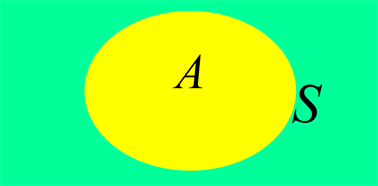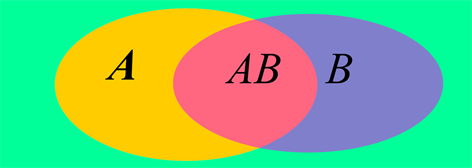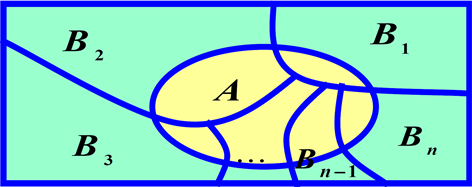﻿ 关于条件概率教学方法的探讨

# 关于条件概率教学方法的探讨Discussion on Teaching Method of Conditional Probability

Abstract: Probability exists everywhere in real life, probability theory and mathematical statistics is an important public basic course in colleges and universities, conditional probability is important knowledge in probability theory and mathematical statistics. In this paper, the author reviews the knowledge from through the example first, then draws out the relevant formula, and gives some derivation, which let the students learn the formula easier.

1. 前言

《概率论与数理统计》是高等院校理工科专业、经管类专业重要的公共基础理论课程，它是研究现实中“随机现象”统计规律性的数学学科，对后续专业课程的深入学习有深远影响。《概率论与数理统计》是数学课程中一个很有特色并且又十分活跃的分支：一方面，它内容丰富，具有自身独特的概念和研究方法；另一方面，它与其他学科有着密切的联系，是近代数学的重要组成部分 。随着科学技术的发展，概率论与数理统计的理论与方法广泛应用于自然科学与社会科学的研究中。例如：它在生物医学、金融、经济管理、工农业生产都具有广泛的应用。

《概率论与数理统计》在大学课程中具有举足轻重的地位，然而，它因理论复杂、应用广而且灵活，长期被看成是一门枯燥难学的课程，从而学生不爱学、难学，教师教学难度也随着增加。因此，研究如何才能提高学生对这门课程的学习兴趣，如何才能提高学生的学习效率与应用能力具有重要的意义。

2. 教学设计

2.1. 条件概率公式

$S=\left\{HH,HT,TH,TT\right\},\text{\hspace{0.17em}}A=\left\{HH,HT,TH\right\},\text{\hspace{0.17em}}B=\left\{HH,TT\right\}.$

$P\left(A\right)=\frac{n\left(A\right)}{n\left(S\right)}=\frac{3}{4},\text{\hspace{0.17em}}P\left(B\right)=\frac{n\left(B\right)}{n\left(S\right)}=\frac{2}{4}=\frac{1}{2}.$Figure 1. Venn diagram (probability of things A and B happening)Figure 2. Venn diagram (probability of occurrence of event A and event B is known)

$P\left(B|A\right)=\frac{P\left(AB\right)}{P\left(A\right)}=\frac{1}{4}.$

$P\left(A|B\right)=\frac{P\left(AB\right)}{P\left(B\right)}=\frac{1}{2}.$

2.2. 乘法公式

$P\left(ABC\right)=P\left(C|AB\right)P\left(AB\right)=P\left(C|AB\right)P\left(B|A\right)P\left(A\right).$

$\begin{array}{c}P\left({A}_{1}{A}_{2}\cdots {A}_{n}\right)=P\left({A}_{n}|{A}_{1}{A}_{2}\cdots {A}_{n-1}\right)P\left({A}_{1}{A}_{2}\cdots {A}_{n-1}\right)\\ =P\left({A}_{n}|{A}_{1}{A}_{2}\cdots {A}_{n-1}\right)P\left({A}_{n-1}|{A}_{1}{A}_{2}\cdots {A}_{n-2}\right)P\left({A}_{1}{A}_{2}\cdots {A}_{n-2}\right)\\ =\cdots \\ =P\left({A}_{n}|{A}_{1}{A}_{2}\cdots {A}_{n-1}\right)P\left({A}_{n-1}|{A}_{1}{A}_{2}\cdots {A}_{n-2}\right)\cdots P\left({A}_{2}|{A}_{1}\right)P\left({A}_{1}\right)\end{array}$.

$P\left({A}_{1}{A}_{2}{\stackrel{¯}{A}}_{3}\right)=P\left({\stackrel{¯}{A}}_{3}|{A}_{1}{A}_{2}\right)P\left({A}_{2}|{A}_{1}\right)P\left({A}_{1}\right)=\frac{t}{r+t+2a}\cdot \frac{r+a}{r+t+a}\cdot \frac{r}{r+t}.$

2.3. 全概率公式

1) ${B}_{i}{B}_{j}=\varnothing$$i\ne j$$i,j=1,\cdots ,n$

2) ${B}_{1}\cup {B}_{2}\cup \cdots \cup {B}_{n}=S$

$P\left(A\right)=P\left(A|{B}_{1}\right)P\left({B}_{1}\right)+P\left(A|{B}_{2}\right)P\left({B}_{2}\right)+\cdots +P\left(A|{B}_{n}\right)P\left({B}_{n}\right).$

$P\left(A\right)=P\left(A{B}_{1}\right)+P\left(A{B}_{2}\right)+\cdots +P\left(A{B}_{n}\right)=P\left(A|{B}_{1}\right)P\left({B}_{1}\right)+P\left(A|{B}_{2}\right)P\left({B}_{2}\right)+\cdots +P\left(A|{B}_{n}\right)P\left({B}_{n}\right).$Figure 3. Schematic diagram of the division of the total probability formula

$P\left(B|\stackrel{¯}{A}\right)=0.8，\text{\hspace{0.17em}}P\left(\stackrel{¯}{B}|A\right)=0.99，\text{\hspace{0.17em}}P\left(A\right)=0.8.$

$P\left(B\right)=P\left(B|A\right)P\left(A\right)+P\left(B|\stackrel{¯}{A}\right)P\left(\stackrel{¯}{A}\right)=0.01×0.8+0.8×0.2=0.168，$

$P\left(A|\stackrel{¯}{B}\right)=\frac{P\left(A\stackrel{¯}{B}\right)}{P\left(\stackrel{¯}{B}\right)}=\frac{P\left(\stackrel{¯}{B}|A\right)P\left(A\right)}{P\left(\stackrel{¯}{B}|A\right)P\left(A\right)+P\left(\stackrel{¯}{B}|\stackrel{¯}{A}\right)P\left(\stackrel{¯}{A}\right)}=\frac{0.99×0.8}{0.832}\approx 0.9519.$

3. 结语

 盛骤, 谢式千, 潘承毅. 概率论与数理统计[M]. 北京: 高等教育出版社, 2008: 2-24.

 王芬. 案例教学法在概率论与数理统计教学中的应用[J]. 高教学刊, 2016(20): 74-75.

 金丹, 熊萍. 案例教学法在《概率论与数理统计》中的教学实践[J]. 科技经济导刊, 2019, 27(36): 103-104.

 郭琴. 关于全概率公式与贝叶斯公式的教学探讨[J]. 科技视界, 2019(11): 247-248.

 陈中明. 全概率公式与贝叶斯公式的启发式教学设计浅谈[J]. 教育教学论坛, 2019(25): 202-203.

 张云霞. 案例教学法在概率论与数理统计教学中的应用[J]. 国际公关, 2019(11): 108.

Top